### A Practical Guide to Univariate Time Series Models with Seasonality and Exogenous Inputs using Finance Data of FMCG Manufacturers

Posted August 21, 2021 by Gowri Shankar  ‐  10 min read

The definition of univariate time series is, a time series that consists of single scalar observations recorded sequentially over equal periodic intervals. i.e An array of numbers are recorded where time is an implicit dimension represented at constant periodicity. Univariate time series models(UTSM) are the simplest models that allow us to forecast the future values by learning the patterns in the sequence of observations recorded. The key elements of these patterns are Seasonality, Trends, Impact Points and Exogenous Variables. There are 3 schemes of pattern identification acts as building block for UTSMs, they are auto regression(OLS), moving averages and seasonality - When they augmented with external data, effectiveness of the model improves significantly.

In this post, we shall study 8 different flavors of UTSMs by using statsmodels package. This post is an introductory guide to use statsmodel package where I focussed more towards the coding aspects rather than the mathematical foundation of the models studied. This is the 4th post on the Time Series Analysis where we studied Structural Time Series through GAMs, Please refer here

### Objective

The objective of this post is to do hands-on coding of univariate time series models for forecasting problems. In this process, we will be covering the following topics

• Yahoo Finance APIs to get stock market data
• Auto Regressive models, Moving Averages and Integrated models
• Seasonality factors and exogenous data

## Introduction

Univariate analysis is a qualitative analysis of single variable changing at certain reference dimension. When the reference dimension is time, we call it univariate time series analysis - Which is the simplest of all time series analysis modeling schemes. When there is only one variable to account, the output or the predicted value is linearly depend on its own previous values. Once they are combined with moving averages, we form a special cases of linear dependents like ARMA to ARIMA etc. Further we can extend this by adding seasonality(SARMA) and exogenous data(SARIMAX) for better outcomes.

In this post, we acquire the stock market day close data of 4 Fast Moving Consumer Goods(FMCG) manufacturers and build our time series models. Our approach is to build a simplest model and improve it step by step by adding various schemes to it. As I mentioned earlier, this is more focussed on statsmodel package usage for time series forecasting rather than studying the nuances of the algorithm behind - an unscholarly attempt for time series.

### Data

Using yfinance package, we download stock market data of

• Unilever, India
• Procter and Gamble, India
• Nestle, India and
• Diageo India

from 1st April, 2020 to 18th August, 2021. We then split the data by 80/20 ratio for train and test. Note, split happens sequentially rather than random choices to ensure the data is Independent and Identically Distributed to avoid leakage or interpolation of trend.

START_DATE = "2020-04-01"
END_DATE = "2021-08-18"
TRAIN_TEST_SPLIT = 0.8

PREDICTION_START_DATE = "2021-05-10"
PREDICTION_END_DATE = "2021-08-18"

import pandas as pd
import matplotlib.pyplot as plt
import seaborn as sns

import warnings
warnings.filterwarnings("ignore")
sns.set()

warnings.filterwarnings('ignore', 'statsmodels.tsa.arima_model.ARMA',
FutureWarning)
warnings.filterwarnings('ignore', 'statsmodels.tsa.arima_model.ARIMA',
FutureWarning)


### Preprocessing

In this section, following activities are accomplished

• Using yfinance package data acquisition is done from NSE, India index for the tickers HINDUNILVR.NS, PGHH.NS, NESTLEIND.NS and MCDOWELL-N.NS
• We are analyzing the day close values, hence the rest of the observations are dropped
• By setting frequency as b, we consider business days of the week as discrete sequence
• Often data is not available for all weekdays due to holidays, imputing for those days are done using forward fill(ffill) scheme
• Train and test data sequence are split by 80/20 ratio
import yfinance
ticker_dict = {
"Unilever": "HINDUNILVR.NS",
"P&G": "PGHH.NS",
"Nestle": "NESTLEIND.NS",
"Diageo": "MCDOWELL-N.NS"
}
tickers = [value for key, value in ticker_dict.items()]

end = END_DATE, interval = "1d", group_by = 'ticker', auto_adjust = True, treads = True)
df_orig = df.copy()

# Day close and drop others
for key, value in ticker_dict.items():
df[key]=df[value].Close[:]
del df[value]

# Set frequency on business days of the week and Forward fill
df = df.asfreq('b').fillna(method='ffill')
df.shape

### Splitting the Data
train_length = int(len(df) * TRAIN_TEST_SPLIT)
df_train = df.iloc[:train_length]
df_test = df.iloc[train_length:]

[*********************100%***********************]  4 of 4 completed


## Forecasting, Simple

Our forecasting section is broadly classified into 3

• Simple Forecast - Auto Regressive and Moving Averages
• Seasonal Forecast
• Integrated Forecast
• Forecast with Exogenous Variables

#### Simple Forecasting

Let us build 2 utility functions, one for training, predicting and storing the results for all the tickers in an efficient way and the second one for plotting the test and predicted data in a grid for easy comprehension.

ARIMA Util

• We use ARIMA API from the statsmodel package
• Train the model for the period starting 1st April, 2020 and ending 7th May, 2021
• Test for the period from 10th May, 2021 to 18th August, 2021
from statsmodels.tsa.arima_model import ARIMA

def arima_util(model_name, model_config, enforce_stationarity=False):

model_output = {}
for key in ticker_dict.keys():
model_output[key] = {}

fit_model = None
predictions_df = None
try:
model = ARIMA(df_train[key], order=model_config)
fit_model = model.fit()
predictions_df = fit_model.predict(start=min(df_test.index), end=max(df_test.index))
model_output[key]["isFit"] = True
except Exception as e:
print(f"Exception Occurred, while training {key} : ", e.__class__)
print(e)
model_output[key]["isFit"] = False

model_output[key]["model"] = fit_model
model_output[key]["predictions"] = predictions_df

return model_output

def plt_predictions(df_actuals, model_predictions, title):
fig, axs = plt.subplots(2, 2, figsize=(30, 20))
fig.suptitle(title, size=32)
row = 0
col = 0
for key in ticker_dict.keys():
predictions = model_predictions[key]
ax = axs[row, col]
actuals = df_actuals[key]
minn = actuals.min()
maxx = actuals.max()
ax.set_ylim(minn - minn * 0.1, maxx + maxx * 0.1)
if predictions["isFit"]:
ax.plot(predictions["predictions"], color="red")
ax.plot(df_actuals[key], color="blue")
ax.set_title(key, size=24)
col += 1
if(col == 2):
row += 1
col = 0

plt.show()


The key parameter of ARIMA API is its order sequence, order sequence determines the selection of model for the training.


The (p,d,q) order of the model for the autoregressive, differences, and moving average components. d is always an integer, while p and q may either be integers or lists of integers.

autoregressive models: AR(p)
moving average models: MA(q)
mixed autoregressive moving average models: ARMA(p, q)
integration models: ARIMA(p, d, q)
seasonal models: SARIMA(P, D, Q, s)

- www.statsmodels.org



### Auto Regressive

Auto regressive models are nothing but a simple linear regression done on the data over time. i.e In regular linear regression, outcome is correlated to the predictors whereas in AR models outcome is dependent on the previous values of its own. AR scheme is a stochastic process that has certain degree of uncertainty due to built-in randomness, makes it never a 100% accurate prediction.They are also Markovian in nature.

auto_regressive_models = arima_util("Auto Regressive", (1, 0, 0))
plt_predictions(df_test[PREDICTION_START_DATE:PREDICTION_END_DATE], auto_regressive_models, "Auto Regressive(AR)")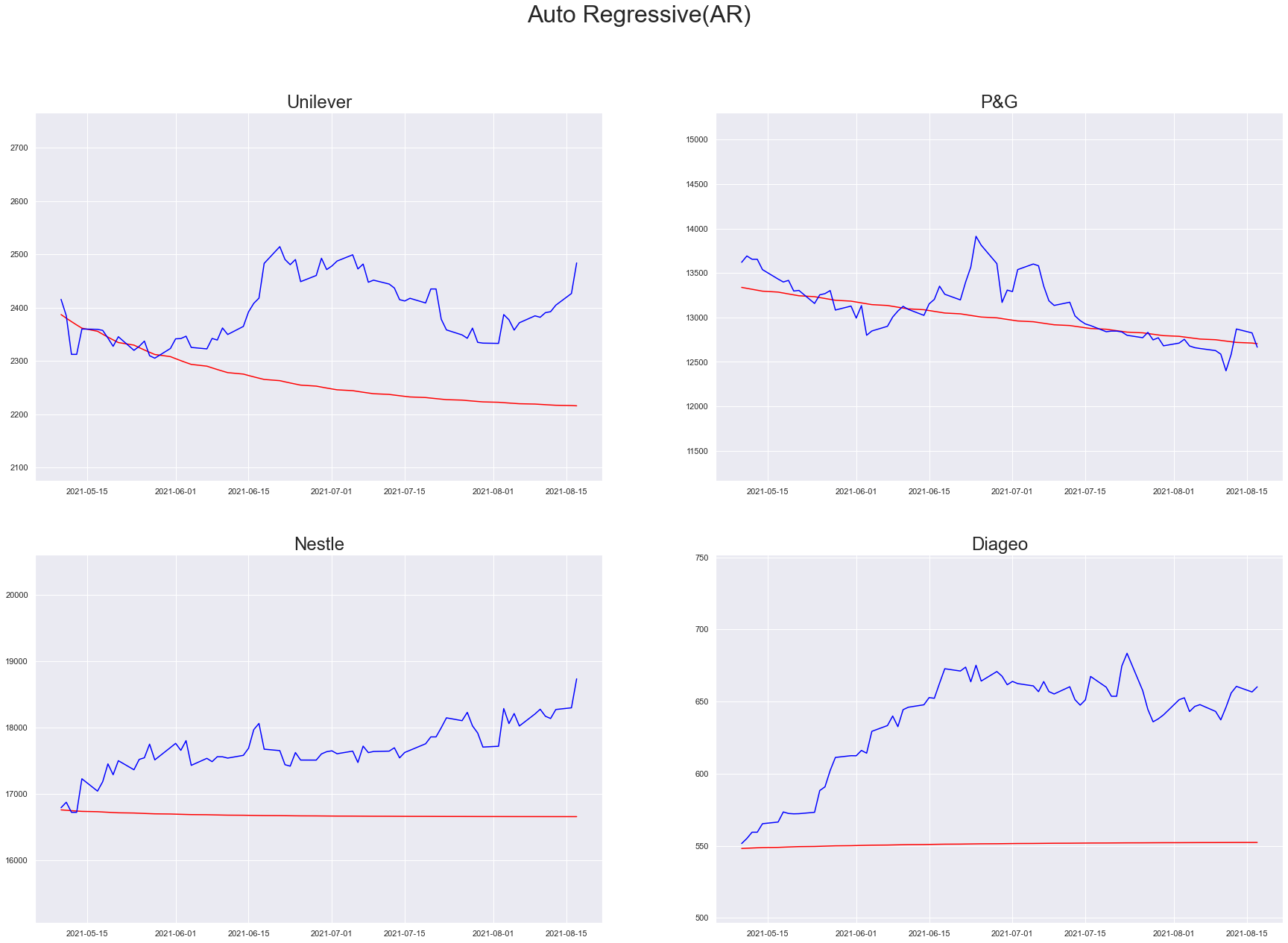### Moving Averages

A simple explanation for Moving Average is the next observation is the mean of past observations. The key difference between AR and MA is, AR part accomplishes the regression of its own lagged values(of the past) and MA accounts for short-run autocorrelation.

moving_averages_models = arima_util("Moving Averages(MA)", (0, 0, 1))
plt_predictions(df_test[PREDICTION_START_DATE:PREDICTION_END_DATE], moving_averages_models, "Moving Averages(MA)")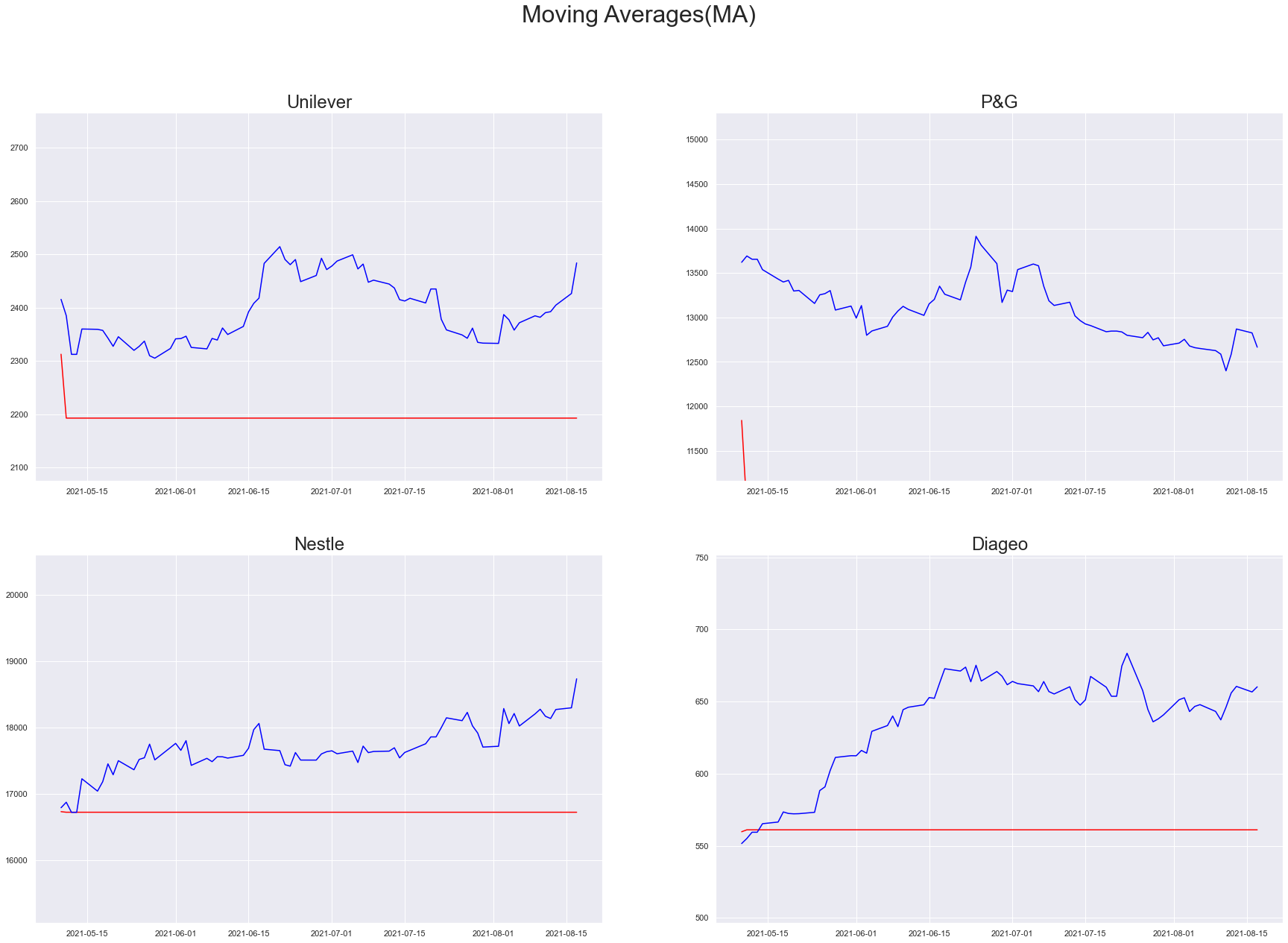### Auto Regressive - Moving Averages(ARMA)

We are studying two closely related ideas ARMA and ARIMA, the key difference between them is the process of differencing. ARMA models are stationary, stationarity in simple terms is a flat looking series with no trend and constant variance over time.


Differencing is where your data has one less data point than the original data set; You’re subtracting
(or moving) a point—a “difference”. For example, given a series Zt you can create a new series
Yi = Zi – Zi – 1.

In calculus, differencing is used for numerical differentiation. The general idea is similar
(e.g. with backwards differencing you are subtracting a point), but the applications are different.
While differencing in calculus still involves “moving” a point, the movement is so that you can
estimate a derivative (i.e. the slope).

- www.statisticshowto.com


arma_models = arima_util("ARMA", (1, 0, 1))
plt_predictions(df_test[PREDICTION_START_DATE:PREDICTION_END_DATE], arma_models, "Auto Regressive Moving Averages(ARMA)")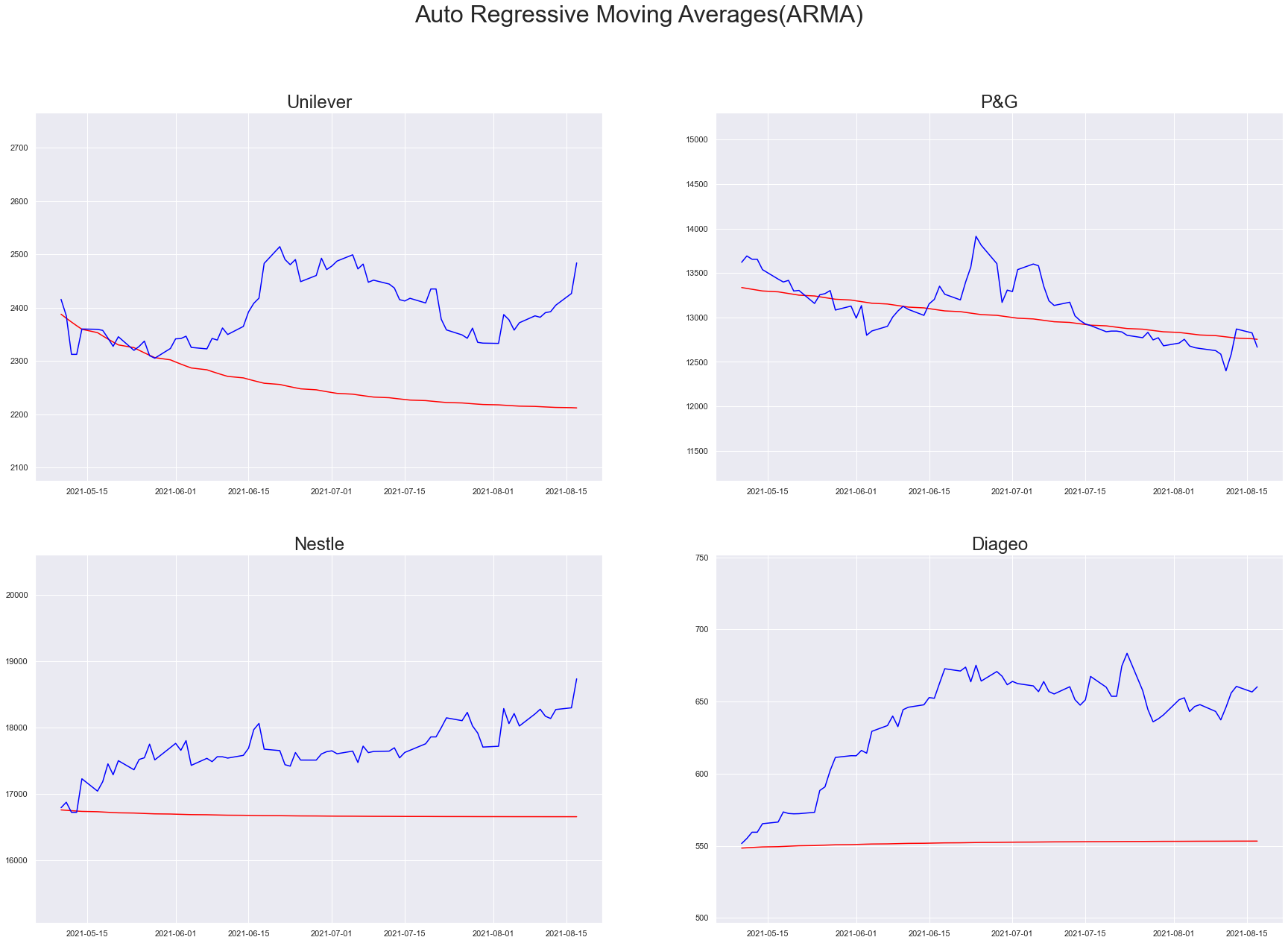## Integrated Model

### ARIMA

The term I in ARIMA represents the measure of non-seasonal differences required to achieve stationarity . In ARMA modes we had the order (p, q) whereas in ARIMA we shall have the order (p, d, q).

arima_models = arima_util("ARIMA", (1, 1, 1))
plt_predictions(df_test[PREDICTION_START_DATE:PREDICTION_END_DATE], arma_models, "Auto Regressive Integrated Moving Averages(ARIMA)")


## Seasonality

Time series data often exhibits seasonal variation and trend, we are extending the ARMA model utility with Seasonal aspect through SARIMAX API. Similar to ARIMA API, we have order sequence that inputs $(p, d, q)$ whereas in SARIMAX along with order, seasonal sequence $s$ with $(P, D, Q, s)$ are fed in.


The (P,D,Q,s) order of the seasonal component of the model for the AR parameters, differences,
MA parameters, and periodicity. Default is (0, 0, 0, 0). D and s are always integers, while P
and Q may either be integers or lists of positive integers.

- www.statisticshowto.com


from statsmodels.tsa.statespace.sarimax import SARIMAX

def sarima_util(model_name, model_config, seasonal_order, exogenous=False, enforce_stationarity=False):

model_output = {}
for key in ticker_dict.keys():
print(f"Training {key}")
model_output[key] = {}
exog = None
exog_test = None
if(exogenous):
keys = ticker_dict.copy()
del keys["P&G"]
exog_keys = keys.keys()
exog = df_train[exog_keys]
exog_test = df_test[PREDICTION_START_DATE:PREDICTION_END_DATE][exog_keys]

fit_model = None
predictions_df = None
try:
model = SARIMAX(df_train[key], order=model_config, seasonal_order=seasonal_order, exog=exog, enforce_stationarity=enforce_stationarity)
fit_model = model.fit()
predictions_df = fit_model.predict(start=min(df_test.index), end=max(df_test.index), exog=exog_test)
model_output[key]["isFit"] = True
except Exception as e:
print("Exception Occurred, while training {key} : ", e.__class__)
print(e)

model_output[key]["isFit"] = False

model_output[key]["model"] = fit_model
model_output[key]["predictions"] = predictions_df

return model_output


### SARMA

# Freeze
sarma_model = sarima_util("SARMA", (1, 0, 1), seasonal_order=(1, 0, 1, 5))
plt_predictions(df_test[PREDICTION_START_DATE:PREDICTION_END_DATE], sarma_model, "Seasonal Auto Regressive Moving Averages(MA)")

Training Unilever

/Users/shankar/dev/tools/anaconda3/envs/od/lib/python3.8/site-packages/statsmodels/base/model.py:566: ConvergenceWarning: Maximum Likelihood optimization failed to converge. Check mle_retvals
warnings.warn("Maximum Likelihood optimization failed to "

Training P&G
Training Nestle
Training Diageo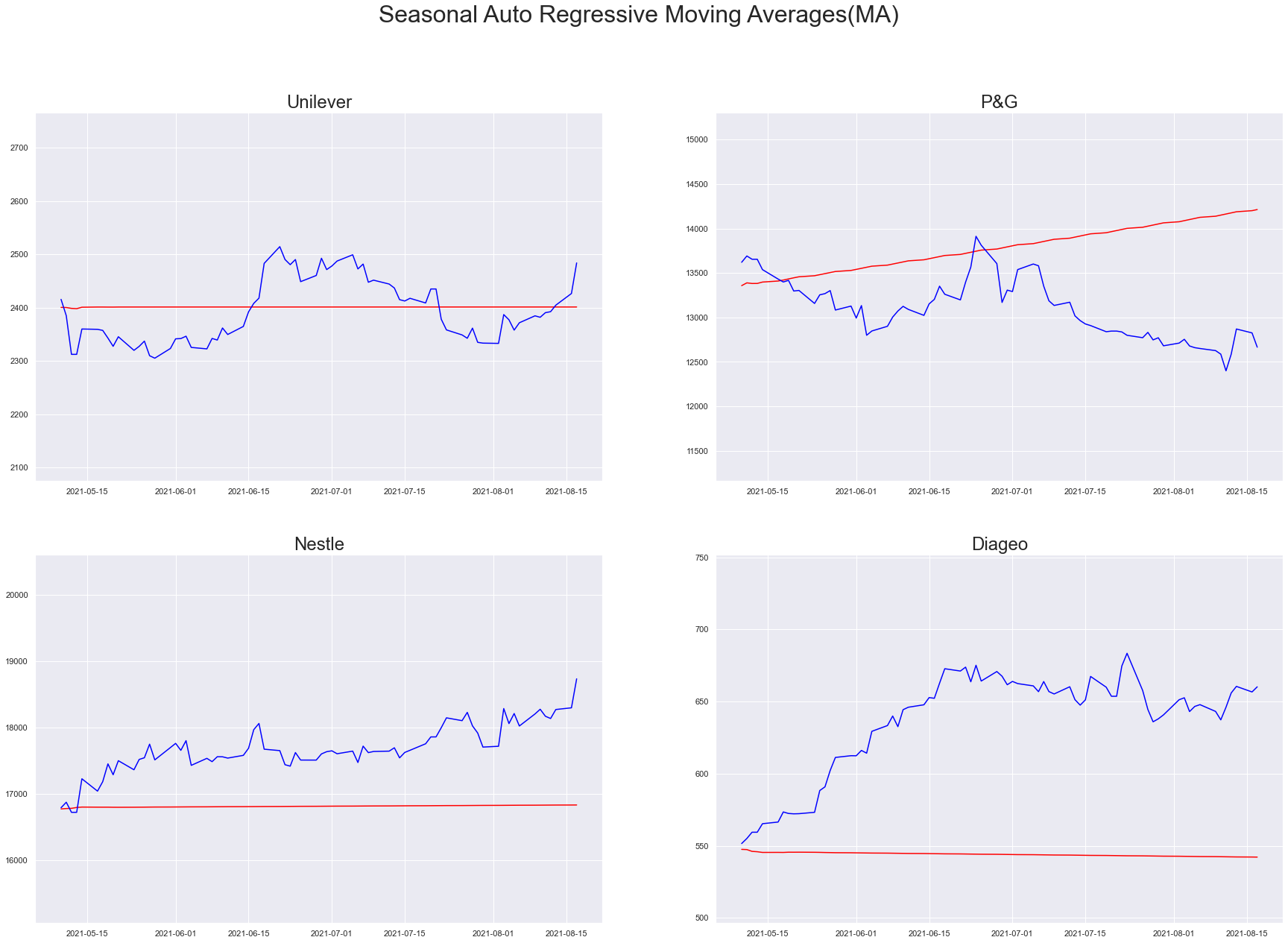### SARIMA

As we add more and more information to the modeling scheme, our prediction plots are showing significant change in the predictability. It is quite evident in SARIMA, tweaking the order and seasonal order hyperparameter is the key activity for convergence.

sarma_model = sarima_util("SARMA", (3, 1, 4), seasonal_order=(3, 1, 2, 5))
plt_predictions(df_test[PREDICTION_START_DATE:PREDICTION_END_DATE], sarma_model, "Seasonal Auto Regressive Moving Averages(MA)")

Training Unilever

/Users/shankar/dev/tools/anaconda3/envs/od/lib/python3.8/site-packages/statsmodels/base/model.py:566: ConvergenceWarning: Maximum Likelihood optimization failed to converge. Check mle_retvals
warnings.warn("Maximum Likelihood optimization failed to "

Training P&G

/Users/shankar/dev/tools/anaconda3/envs/od/lib/python3.8/site-packages/statsmodels/base/model.py:566: ConvergenceWarning: Maximum Likelihood optimization failed to converge. Check mle_retvals
warnings.warn("Maximum Likelihood optimization failed to "

Training Nestle

/Users/shankar/dev/tools/anaconda3/envs/od/lib/python3.8/site-packages/statsmodels/base/model.py:566: ConvergenceWarning: Maximum Likelihood optimization failed to converge. Check mle_retvals
warnings.warn("Maximum Likelihood optimization failed to "

Training Diageo

/Users/shankar/dev/tools/anaconda3/envs/od/lib/python3.8/site-packages/statsmodels/base/model.py:566: ConvergenceWarning: Maximum Likelihood optimization failed to converge. Check mle_retvals
warnings.warn("Maximum Likelihood optimization failed to "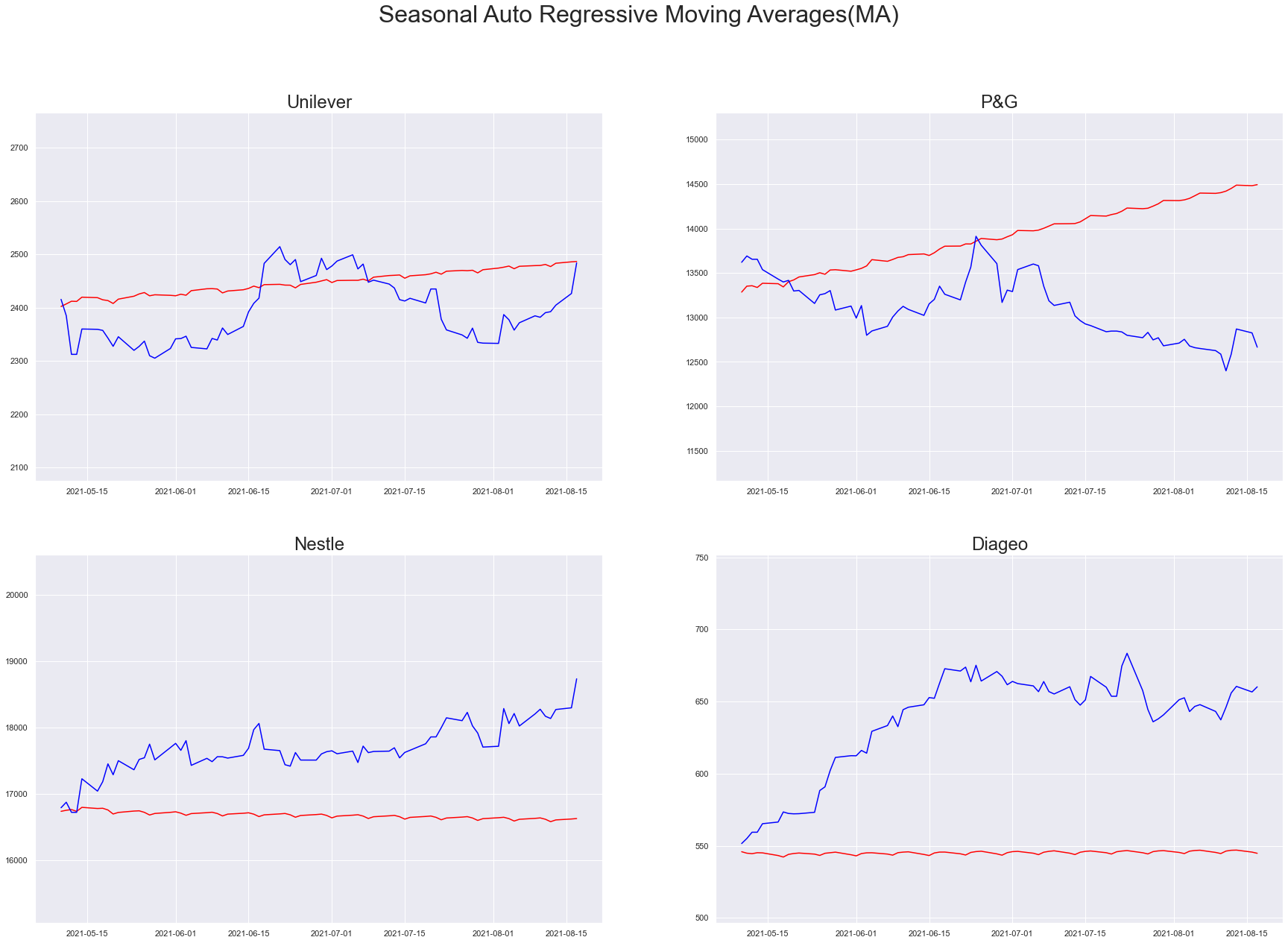## Exogenous Model(X)

Exogenous models learn patterns from the external data, In this study we feed Unilever, Nestle and Diageo data as exogenous ones to predict P&G’s outcomes. In this section we shall compare the predictability of various combinations of model schemes.

def exogenous_util(ticker, order, seasonal_order=None, enforce_stationarity=False):
model_output = {}
print(f"Training {ticker}")
model_output = {}

keys = ticker_dict.copy()
del keys["P&G"]
exog_keys = keys.keys()
exog = df_train[exog_keys]
exog_test = df_test[PREDICTION_START_DATE:PREDICTION_END_DATE][exog_keys]

fit_model = None
predictions_df = None
try:
model = SARIMAX(df_train[ticker], order=order, seasonal_order=seasonal_order, exog=exog, enforce_stationarity=enforce_stationarity)
fit_model = model.fit()
predictions_df = fit_model.predict(start=min(df_test.index), end=max(df_test.index), exog=exog_test)
model_output["isFit"] = True
except Exception as e:
print("Exception Occurred, while training {key} : ", e.__class__)
print(e)

model_output["isFit"] = False

model_output["model"] = fit_model
model_output["predictions"] = predictions_df

return model_output


### ARIMAX

We are also enforcing stationarity in these models to notice how better the models are converging as we add more and more information.

armax_models = exogenous_util("P&G", (3, 0, 4), enforce_stationarity=True)
arimax_models = exogenous_util("P&G", (3, 1, 4), enforce_stationarity=True)

Training P&G

/Users/shankar/dev/tools/anaconda3/envs/od/lib/python3.8/site-packages/statsmodels/base/model.py:566: ConvergenceWarning: Maximum Likelihood optimization failed to converge. Check mle_retvals
warnings.warn("Maximum Likelihood optimization failed to "

Training P&G

/Users/shankar/dev/tools/anaconda3/envs/od/lib/python3.8/site-packages/statsmodels/base/model.py:566: ConvergenceWarning: Maximum Likelihood optimization failed to converge. Check mle_retvals
warnings.warn("Maximum Likelihood optimization failed to "


plt.figure(figsize=(20, 5))
armax_models["predictions"].plot(color="red", label="ARMAX")
arimax_models["predictions"].plot(color="green", label="ARIMAX")
df_test["P&G"].plot(color="blue")
plt.legend()
plt.title("P&G Actual vs ARMAX, ARIMAX", size=32)
plt.show()
# plt_predictions(df_test[PREDICTION_START_DATE:PREDICTION_END_DATE], armax_models, "Auto Regressive Moving Averages(MA)")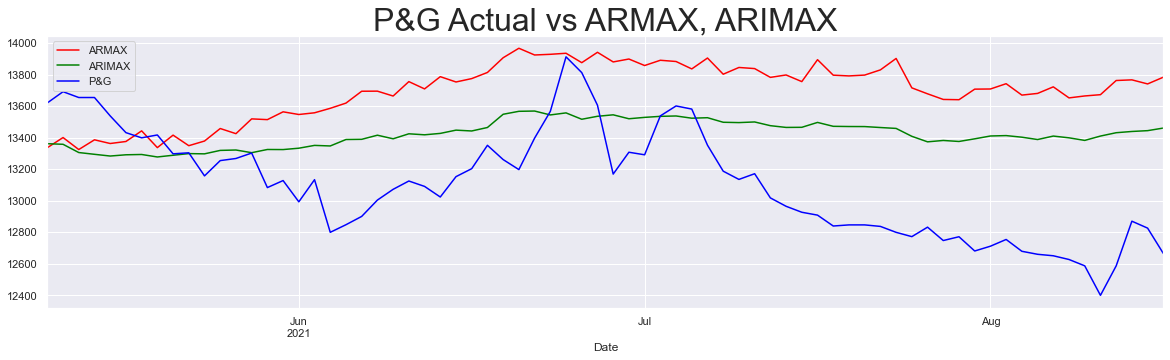### SARIMAX

# Freeze
sarmax_model = exogenous_util("P&G", (3, 0, 4), seasonal_order=(3, 0, 2, 5), enforce_stationarity=True)
sarimax_model = exogenous_util("P&G", (3, 1, 4), seasonal_order=(3, 1, 2, 5), enforce_stationarity=True)
print(sarmax_model.keys())

Training P&G

/Users/shankar/dev/tools/anaconda3/envs/od/lib/python3.8/site-packages/statsmodels/base/model.py:566: ConvergenceWarning: Maximum Likelihood optimization failed to converge. Check mle_retvals
warnings.warn("Maximum Likelihood optimization failed to "

Training P&G

/Users/shankar/dev/tools/anaconda3/envs/od/lib/python3.8/site-packages/statsmodels/base/model.py:566: ConvergenceWarning: Maximum Likelihood optimization failed to converge. Check mle_retvals
warnings.warn("Maximum Likelihood optimization failed to "

dict_keys(['isFit', 'model', 'predictions'])



plt.figure(figsize=(20, 10))
sarmax_model["predictions"].plot(color="red", label="SARMAX")
sarimax_model["predictions"].plot(color="green", label="SARIMAX")
armax_models["predictions"].plot(color="yellow", label="ARMAX")
arimax_models["predictions"].plot(color="magenta", label="ARIMAX")
df_test["P&G"].plot(color="blue", label="Actual")
plt.legend()
plt.title("Comparison of Seasonal, Integrated and Auto Regressive Models for P&G Data", size=32)

plt.show()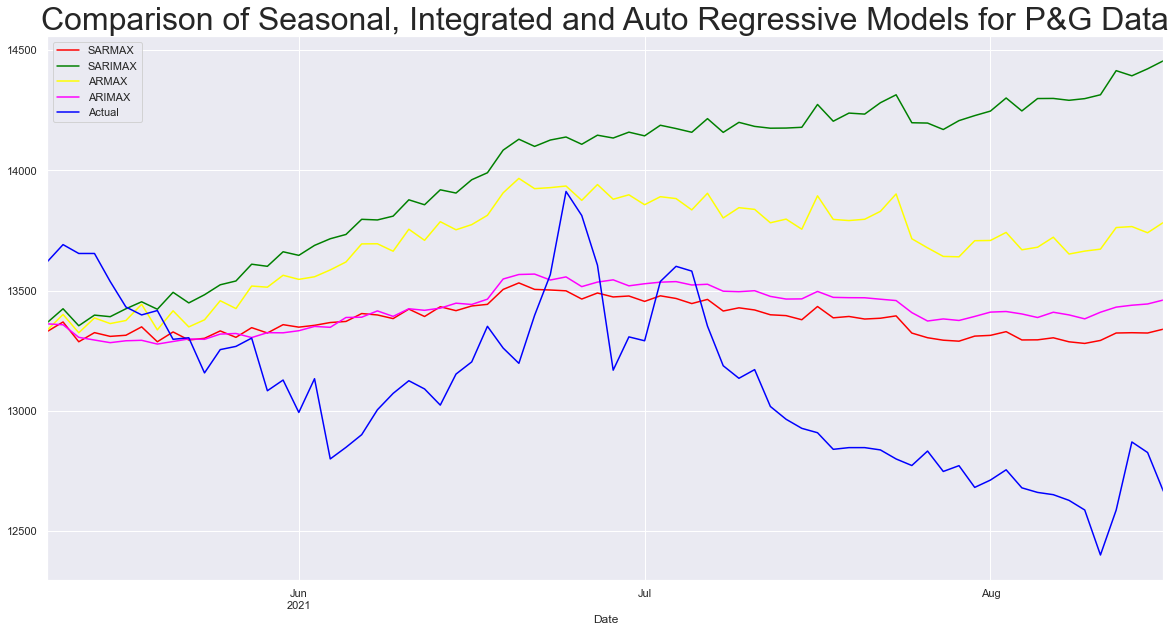## Conclusion

In this post we studied how to use statsmodel package by explore 8 different model types. We also visualize the outcomes graphically, the models we studied from Auto Regressive(AR) to Seasonal Auto Regressive Integrated Moving Average with Exogenous Data(SARIMAX) equip us with understanding and practical implementation of Univariate Time Series analysis and forecasting.